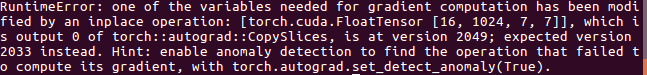# RuntimeError: one of the variables needed for gradient computation has been modified by an inplace operation: [torch.cuda.FloatTensor [16,1024, 7, 7]] which is output 0 of torch::autograd::CopySlices, is at version 2049; expected version 2033 instead

In Depth-wise convolution layer of MobileNet-v1, each channel of the input is convolved with a kernel with channel length = 1, then we use groups = in_channels in Conv2d to produce 1-channel kernels (in_channels = out_channels in depth-wise convolution). In the paper MoBiNet (https://arxiv.org/abs/1907.12629), the author define the term K-dependencyFrom what I understand, this means that each output is now the activation of the sum of the convolution of each corresponding 1-channel kernel with channels in the same group of the inputs.
For example, we have Input with 4 channels C1, C2, C3, C4, then we seperate them into 2 groups, group 1 has C1, C2 and group 2 contains C3, C4. After that we have 4 1-channel kernels K1, K2, K3, K4. Then the output will be
O1 = Activation(C1 K1 + C2 K1),
O2 = Activation(C1 K2 + C2 K2),
O3 = Activation(C3 K3 + C4 K3),
O4 = Activation(C3 K4 + C4 K4)
(* is the convolution operation)
Here is my way to solve the problem:
For i number of params in a group:
Set y = input
Output = input*weight
For each group:
Swap the position (1 to 0, 2 to 1, etc…)

I also incorporate the mid block as in the paper and here is the code:

``````import torch
import torch.nn as nn

class MidBlock(nn.Module):
def __init__(self, inp, oup, stride):

super(MidBlock, self).__init__()
self.stride = stride
self.inp = inp
self.oup = oup
self.conv_dw = nn.Conv2d(inp, inp, 3, stride, 1, groups=inp, bias=False)
self.activate = nn.Sequential(
nn.PReLU(),
nn.BatchNorm2d(inp)
)

self.block = nn.Sequential(
nn.Conv2d(inp, inp, 1, 1, 0, bias=False),
nn.PReLU(),
nn.BatchNorm2d(inp)
)

self.pw = nn.Sequential(
nn.Conv2d(inp, oup, 1, 1, 0, bias = False),
nn.PReLU(),
nn.BatchNorm2d(oup)
)

self.down2 = nn.Conv2d(in_channels=inp, out_channels=oup, kernel_size=1, stride=1, padding=0, bias=False)
self.down1 = nn.Conv2d(in_channels=inp, out_channels=inp, kernel_size=1, stride=stride, padding=0, bias=False)
def forward(self, x):
temp = x
param_in_group = int(self.inp/8)
if self.stride != 1:
temp = self.down1(temp)
out = 0
#out = out.cuda()
for j in range(int(param_in_group)):
y = x
out += self.conv_dw(x)
for k in range(8):
x[:,param_in_group*k:param_in_group*(k+1)-2,:,:] = y[:,param_in_group*k+1:param_in_group*(k+1)-1,:,:]
x[:,param_in_group*(k+1)-1,:,:] = y[:,param_in_group*k,:,:]

out  = self.activate(out)
out += temp
out  = self.block(out)
temp = out
out  = self.pw(out)
if self.inp != self.oup:
temp = self.down2(temp)
out += temp
return out

class Net(nn.Module):
def __init__(self):
super(Net, self).__init__()
self.conv_bn = nn.Sequential(
nn.Conv2d(in_channels=3, out_channels=32, kernel_size=3, stride=2, padding=1, bias=False),
nn.BatchNorm2d(32),
nn.PReLU()
)
self.block1  = MidBlock(32, 64, 1)
self.block2  = MidBlock(64, 128, 2)
self.block3  = MidBlock(128, 128, 1)
self.block4  = MidBlock(128, 256, 2)
self.block5  = MidBlock(256, 256, 1)
self.block6  = MidBlock(256, 512, 2)
self.block7  = MidBlock(512, 512, 1)
self.block8  = MidBlock(512, 512, 1)
self.block9  = MidBlock(512, 512, 1)
self.block10 = MidBlock(512, 512, 1)
self.block11 = MidBlock(512, 512, 1)
self.block12 = MidBlock(512, 1024, 2)
self.block13 = MidBlock(1024, 1024, 1)
self.avgPool = nn.AvgPool2d(7)
self.fc = nn.Linear(1024, 2)

def forward(self, x):
out = self.conv_bn(x)
out = self.block1(out)
out = self.block2(out)
out = self.block3(out)
out = self.block4(out)
out = self.block5(out)
out = self.block6(out)
out = self.block7(out)
out = self.block8(out)
out = self.block9(out)
out = self.block10(out)
out = self.block11(out)
out = self.block12(out)
out = self.block13(out)
out = self.avgPool(out)
out = out.view(-1,1024)
out = self.fc(out)
return out
``````

but later I encounter this problem in backward passso how should I fix it?